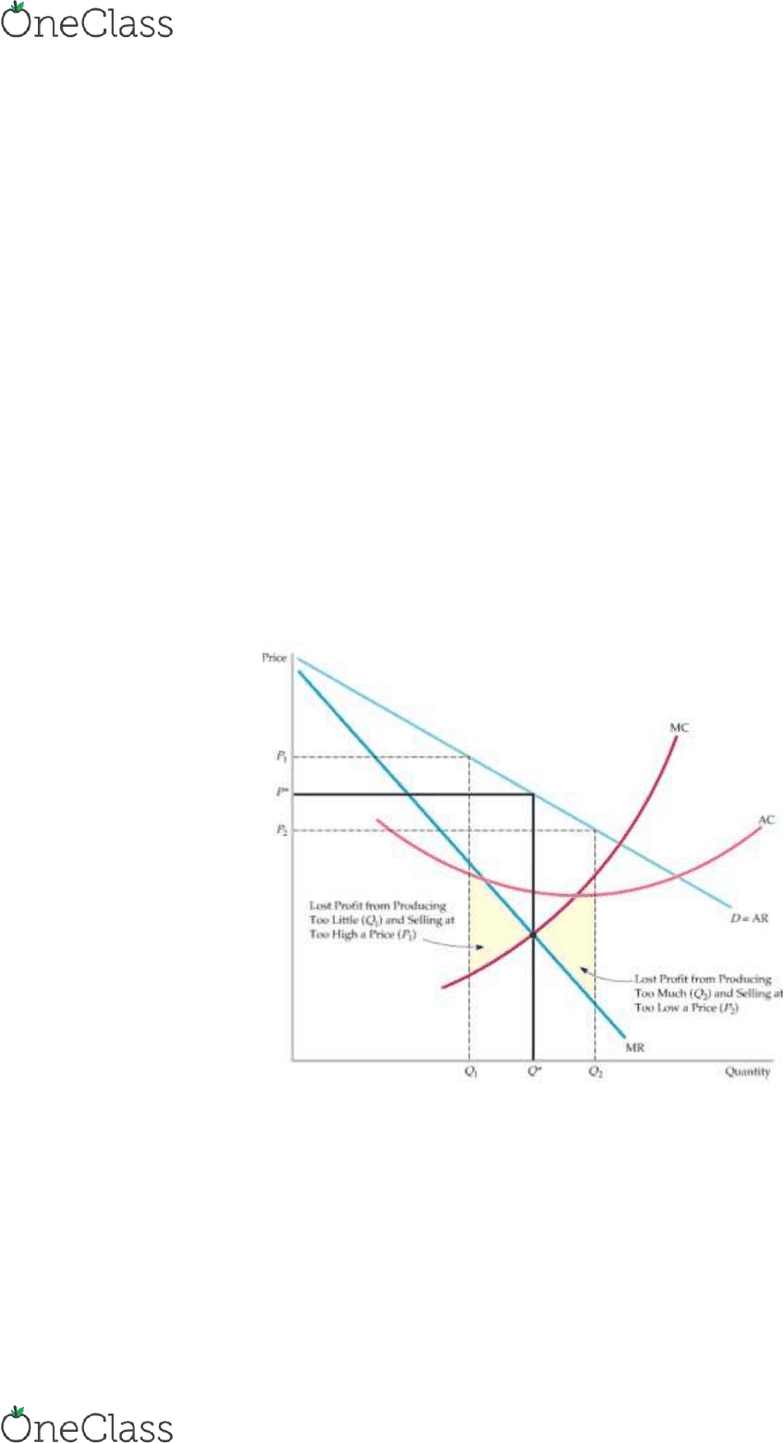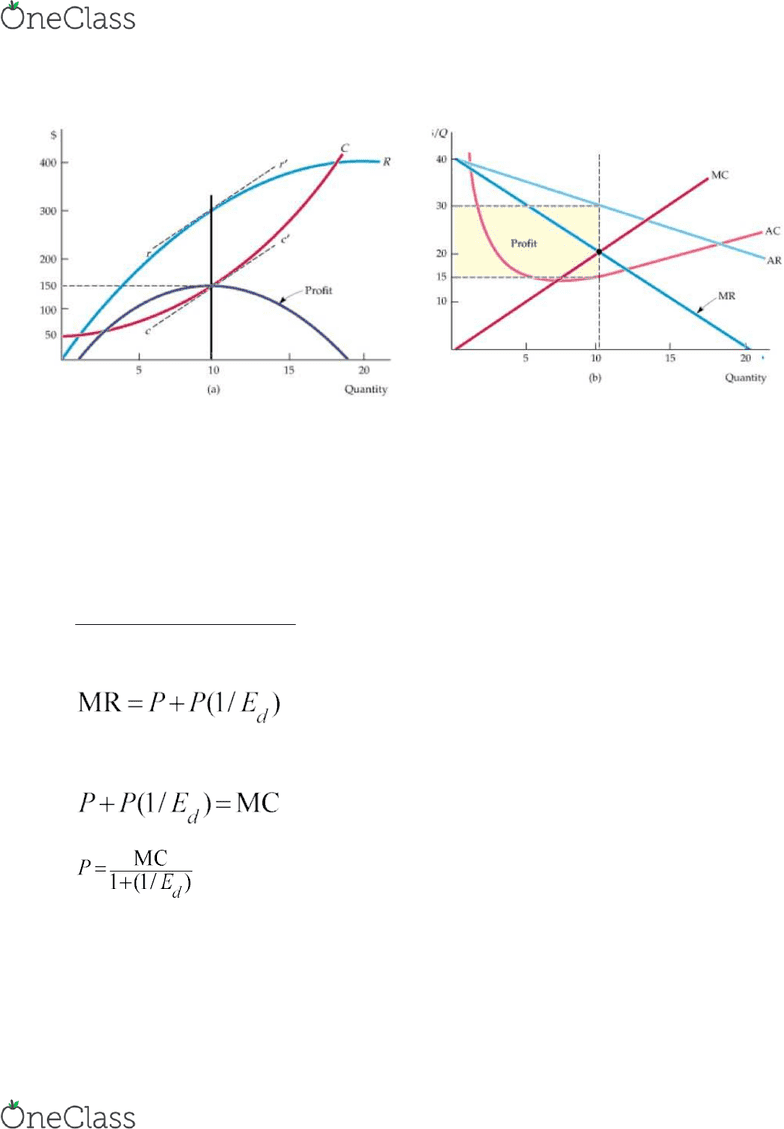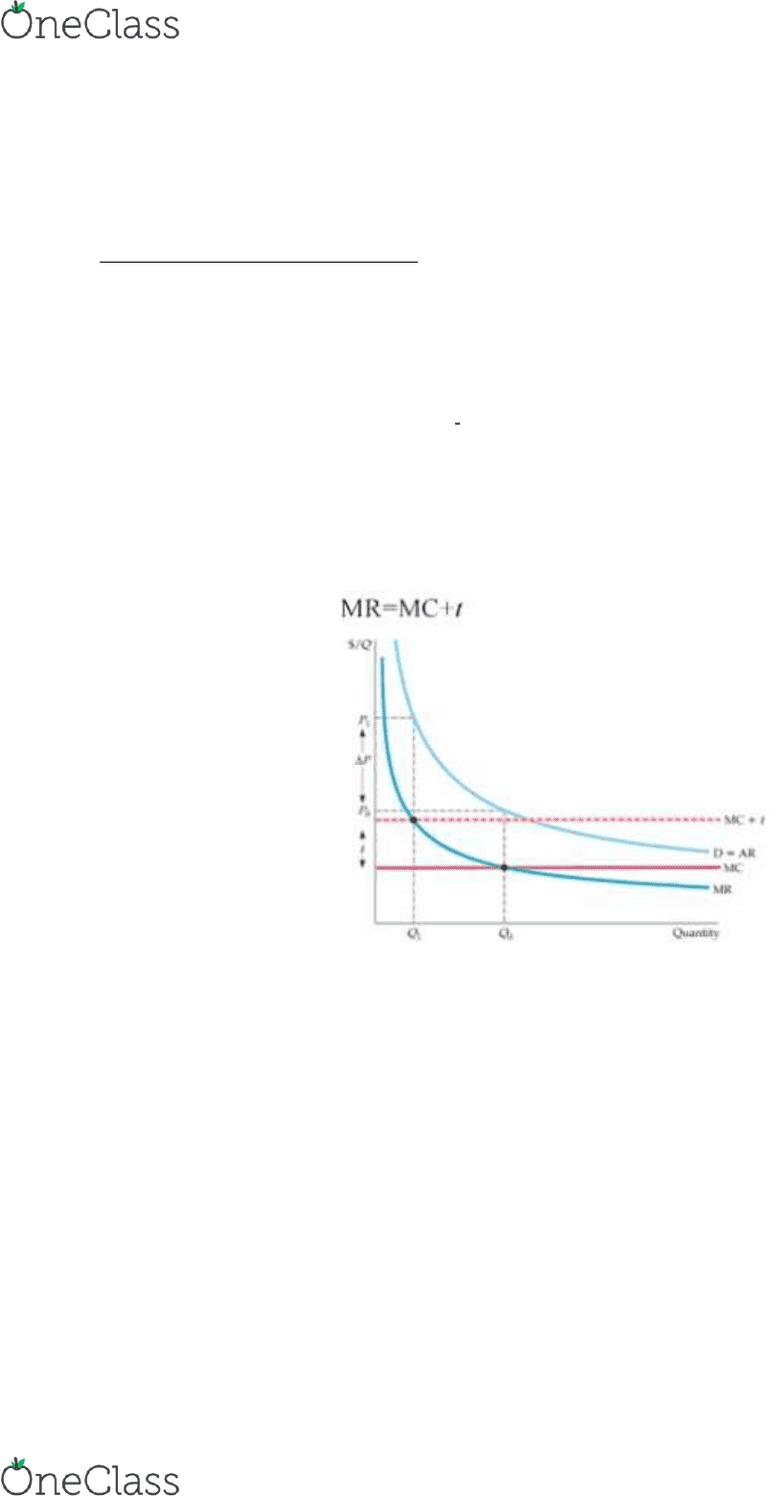# ECON201 Lecture Notes - Lecture 10: Economic Equilibrium, Price Ceiling, Monopsony

33 views10 pages
School
Department
Course
ProfessorECON 221 – Chapter #10 Notes
A Monopoly is the only supplier of a good for which there is no close substitute.
A monopoly’s output is the market output, and the demand curve a monopoly
faces is the market demand curve.
Unlike a competitive firm, a monopolist can set its price.
The monopolist’s average revenue curve is also the market demand curve .
 MR is twice the slope of the demand curve (it is steeper). MR and AR hit the vertical axis
at the same point
 The MR curve hits the horizontal (quantity) axis at half the quantity as the demand curve.
As a matter of fact:
For all linear demand functions with the form:
P(q)= a-bq  MUST BE DERIVED FOR P not Q
 MR=a-2bq
At Q*, MR = MC.  Profit maximizing condition
If the firm produces Q1—it sacrifices some profit because the extra revenue exceeds
the cost of producing them.
Increase Q* to Q2 would reduce profit because the additional cost would exceed the
additional revenue.
find more resources at oneclass.com
find more resources at oneclass.com
Unlock document

This preview shows pages 1-3 of the document.
Unlock all 10 pages and 3 million more documents.

Already have an account? Log inMR is the slope of the total revenue curve, and MC is the slope of the total cost curve.
The profit-maximizing output is Q* = 10, the point where MR=MC (i.e., slopes of the
total revenue and total cost curves are equal).
The profit per unit is \$15, the difference between average revenue and average cost.
Because 10 units are produced, total profit is \$150
A Rule of Thumb for Pricing
We want to translate MR=MC into a rule of thumb that can be easily applied in practice.
Because the firm’s objective is to maximize profit, we can set MR=MC
Recall
If demand is elastic, TR increases when price falls.  Since demand is elastic when price
falls then Qd will increase by more than price falls thus TR increases
find more resources at oneclass.com
find more resources at oneclass.com
Unlock document

This preview shows pages 1-3 of the document.
Unlock all 10 pages and 3 million more documents.

Already have an account? Log inIf demand is inelastic, TR decreases when price falls.  Since demand is inelastic when
price falls then Qd will increase by LESS than price falls thus TR decreases
When q increases, p falls, then
1. If demand is elastic, MR is positive
2. If demand is inelastic, MR is negative
3. If demand is unitary elastic, MR is zero
A monopolist has no supply curve —i.e., there is no one-to-one relationship between
price and quantity produced.
The Effect of a Tax
Suppose a specific tax of t dollars per unit is levied, so that the monopolist must pay t
dollars to the government for every unit it sells.
With a tax t per unit, the firm’s effective marginal cost is increased by the amount t to
MC + t. In this example, the increase in price ΔP is larger than the tax t.
Observations
MC shifts up to MC+t
Intersection corresponds to output level q1
Difference between P1 and P0 is caused by the tax
Increase in P is larger than the amount in tax
In this case, the MC happens to be constant
Vertical distance would be t, this is the effect of the tax
find more resources at oneclass.com
find more resources at oneclass.com
Unlock document

This preview shows pages 1-3 of the document.
Unlock all 10 pages and 3 million more documents.

Already have an account? Log in

# Get access

Grade+
\$10 USD/m
Billed \$120 USD annually
Homework Help
Class Notes
Textbook Notes
40 Verified Answers
Study Guides
1 Booster Class
Class+
\$8 USD/m
Billed \$96 USD annually
Homework Help
Class Notes
Textbook Notes
30 Verified Answers
Study Guides
1 Booster Class